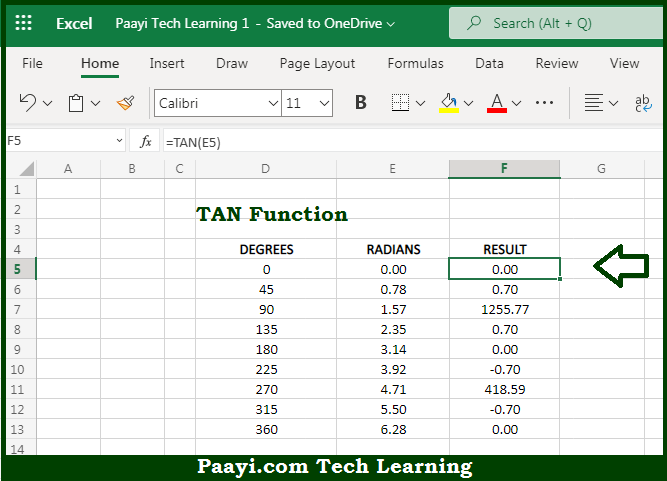# Learn How to Use Microsoft Excel TAN Function

Written by | 0 Comments | 752 Views

In this article, you will learn how to use the Microsoft Excel TAN function and its prime function in Microsoft Excel. You will also get to know the Microsoft Excel TAN function return value and syntax with the help of some examples.

Microsoft Excel TAN Function

The main purpose of the Microsoft Excel TAN function is to get the tangent of the angle. That implies, with the help of the TAN function you can able to return the tangent of an angle provided in the radians. In geometry, the tangent of an angle returns the ratio of a right triangle's opposite side over its adjacent side. You need to multiply the angle by PI()/180 or use the RADIANS function to convert to radians, to supply an angle to TAN in degrees. So, with the help of the TAN function, you can able to get the tangent of an angle provided in the radians.

Return Value of TAN Function

The return value will be the tangent value.

Syntax of TAN Function

=TAN(number)

Where the arguments:

• number: This is the angle in radians of which you want to get the tangent value.

## How to Use Microsoft Excel TAN Function?So we know that Microsoft Excel TAN function you can able to get the tangent of the angle. That implies, with the help of the TAN function you can able to return the tangent of an angle provided in the radians. In geometry, the tangent of an angle returns the ratio of a right triangle's opposite side over its adjacent side. You need to multiply the angle by PI()/180 or use the RADIANS function to convert to radians, to supply an angle to TAN in degrees. So, with the help of the TAN function, you can able to get the tangent of an angle provided in the radians.